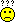programming forums Java Mobile Certification Databases Caching Books Engineering Micro Controllers OS Languages Paradigms IDEs Build Tools Frameworks Application Servers Open Source This Site Careers Other Pie Elite all forums
this forum made possible by our volunteer staff, including ...
Marshals:
• Tim Cooke
• Campbell Ritchie
• Ron McLeod
• Liutauras Vilda
• Jeanne Boyarsky
Sheriffs:
• Junilu Lacar
• Rob Spoor
• Paul Clapham
Saloon Keepers:
• Tim Holloway
• Tim Moores
• Jesse Silverman
• Stephan van Hulst
• Carey Brown
Bartenders:
• Al Hobbs
• Piet Souris
• Frits Walraven

Problem with String

Greenhorn
Posts: 12
••Number of slices to send:
Optional 'thank-you' note:
••Hi,
public class TestString
{
public static void main(String[] args)
{
String s="java";
String s1="ja";
String s2="va";
String s3="ja"+"va";
String s4=s1+s2;
String s5=s+"";
System.out.println(s==s3);// output true
System.out.println(s==s4);// output false
System.out.println(s==s5);// output true
}
}

Plz, explain me(in detail) why I'm getting this output.
senthil

Greenhorn
Posts: 24
••Number of slices to send:
Optional 'thank-you' note:
••the only thing i can guess here is if u initialize the String statically then the compiler does know that it can optimize. but if u initialize a String from other String variables it doesnt. its very ad hoc answer i know.

senthil kumaar
Greenhorn
Posts: 12
••Number of slices to send:
Optional 'thank-you' note:
••Ok vivek, if that is the case then
String s="java";
String s5=s+"";
System.out.println(s==s5); // output true

then the o/p of this should be false, but it is giving true.[This message has been edited by senthil kumaar (edited May 04, 2001).]

vivek bawge
Greenhorn
Posts: 24
••Number of slices to send:
Optional 'thank-you' note:
••Now its very clear to the compiler that adding "" to a string variable isnt going to change it. I think they should have put that much intelligence in the compiler,
-Vivek

Ranch Hand
Posts: 195
••Number of slices to send:
Optional 'thank-you' note:
••Senthil, I pasted your code and compiled, and got slightly different output: the final line (s==s5) returns false for me.
That I understand; what I don't understand is why (s==s3) is returning true. s and s3 are not the same objects and neither has been assigned to point to the other's location in memory. s.equals(s3) should return true, but not s==s3.

Ranch Hand
Posts: 48
••Number of slices to send:
Optional 'thank-you' note:
••Hi Senthil,
This is what I got!
<code>
class Flo1
{
public static void main(String[] args)
{
String s1 = "Java";
String s2 = "Fortran";
String s3 = "JavaFortran";
String s4 = "Java" + "Fortran";
String s5 = s1 + s2;
String s6 = s1+"";
System.out.println(s3.equals(s4));
System.out.println(s3==s4);
System.out.println(s3.equals(s5));
System.out.println(s3==s5);
System.out.println(s1.equals(s6));
System.out.println(s1==s6);
}
}
</code>
The output was:
true
true
true
false
true
false
Explains it? Probably!
Rahul

Ranch Hand
Posts: 147
••Number of slices to send:
Optional 'thank-you' note:
••hi senthil
1. String s3 = "ja" + "va";
here the Strings are which are computed by constant expressions are computed at compile time and treated as if they were literals ... hence this evaluates to true.
2. String s4 = s1 + s2;
here the Strings computed at runtime are newly created and distinct ..... hence this evaluates to false
3. String s5 = s + " "; (notice the space within the quotes)
here too the Strings computed at runtime are newly created and distinct ..... hence this evaluates to false
Now if you want the checks for s4 and s5 to evaluate to true the the newly created Strings have to be interned so as to share the same references. This can be done using the intern() method of the String classThe harder u try the luckier u get